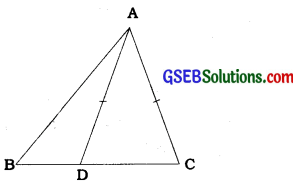# GSEB Class 9 Maths Notes Chapter 7 Heron’s Formula

This GSEB Class 9 Maths Notes Chapter 7 Heron’s Formula covers all the important topics and concepts as mentioned in the chapter.

## Heron’s Formula Class 9 GSEB Notes

Triangle:

• A closed figure formed by three intersecting lines is called a triangle.
• A triangle has three sides, three angles and three vertices. Triangle ABC is denoted as ∆ABC. In ∆ABC, line segments AB, BC and CA are its sides; ∠A, ∠B and ∠C are its angles and A, B and C are its vertices.

Congruent figures:
Congruent means equal in all respect or figures whose shapes and sizes are both the same. Two circles having the Same radius, two squares having the same side and two equilateral triangles having the same side are always congruent.

Correspondence between triangles:
There can be six one-one correspondences between ∆ABC and ∆XYZ. They are ABC ↔ XYZ, ABC XZY, ABC ↔ YXZ, ABC YZX, ABC ZXY, ABC o ZYX. For the correspondence ABC ↔ XYZ between ∆ABC and ∆XYZ, their corresponding parts and vertices are as below:

• Vertices: A ↔ X, B ↔ Y, C ↔ Z
• Sides: AB ↔ XY, BC ↔ YZ, CA ↔ ZX
• Angles: ZA ↔ ZX, ZB ↔ ZY, ZC ↔ ZZ.

Congruence of triangles:
Two triangles are congruent if for a given correspondence the sides and angles of one triangle are equal to the corresponding sides and angles of the other triangle.

For the given correspondence ABC ↔ XYZ between ∆ABC and ∆XYZ, if all their corresponding parts are equal, i.e., ∠A = ∠X, ∠B = ∠Y, ∠C = ∠Z, AB = XY, BC = YZ and CA = ZX, then it is said that the correspondence ABC ↔ XYZ is congruence and the triangles are said to be congruent for that correspondence. Symbolically, it is expressed as ∆ABC ≅ ∆ XYZ.

If the correspondence ABC ↔ XYZ between ∆ABC and ∆XYZ is a congruence, it is not necessary that the other five correspondence are also congruence. Hence, while stating the congruence, the correspondence should be followed strictly (written correctly).

Criteria for congruence of triangles:
Axiom 7.1 (SAS congruence rule): Two triangles are congruent if two sides and the included angle of one triangle are equal to two sides and the included angle of the . other triangle.

Theorem 7.1 (ASA congruence rule):
Two triangles are congruent if two angles and the included side of one triangle are equal to two angles and the included side of the other triangle.

AAS congruence rule:
Two triangles are congruent if any two pairs of angles and one pair of corresponding sides are equal.

CPCT:
The congruence of two triangles can be proved by the equality of any three parts in which at least one is a side. The other three parts of the congruent triangles are also equal. In short form, it is expressed by CPCT which stands for ‘corresponding parts of congruent triangles’.

Example 1:
In the given figures,
OA = OB and OD = OC.Show that
(i) ∆AOD≅BOC and
(I) In ∆AOD and ∆BOC,
Given
OA = OB
OD = OC
Also, since ∠AOD and ∠BOC form a pair of vertically opposite angles, we have ∠AOD = ∠BOC.
So, ∆AOD ≅ ∆BOC (By the SAS congruence rule)

(ii) In congruent triangles AOD and BOC, the other corresponding parts are also equal.
So, ∠OAD = ∠OBC and these form a pair of alternate angles for line segments AD and BC and their transversal AB.Example 2:
AB is a line segment and line l is its perpendicular bisector. If a point P lies on l, show that P is equidistant from A and B.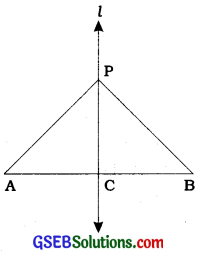Line l ⊥ AB and passes through C which is the midpoint of AB (see the figure).
In ∆PCA and ∆PCB,
AC = BC (C Is the midpoint of AB.)
∠PCA = ∠PCB = 900 (Given)
PC = PC (Common)
So, ∆PCA ≅ ∆PCB (SAS rule)
∴ PA = PB (CPCT)
Thus, P is equidistant from A and B.

Example 3:
Line segment AB is parallel to another line segment CD. O is the midpoint of AD (see in the given figure). Show that
(i) ∆ AOB ≅ ∆DOC
(ii) O is also the midpoint of BC.(i) In ∆AOB and ∆DOC,
∠ABO = ∠DCO (Alternate angles as AB II CD and BC is < the transversal.)
∠AOB = ∠DOC (Vertically opposite angles)
OA = OD (Given)
∴ ∆AOB ≅ ∆DOC (AAS rule)

(ii) Then, OB = OC (CPCT)
So, O is the midpoint of BC.

Some properties of a triangle:
Theorem 7.2:
Angles opposite to equal sides of an isosceles triangle are equal.

Theorem 7.3:
The sides opposite to equal angles of a triangle are equal.
Theorem 7.2 and theorem 7.3 are converse of each other.

Equilateral triangle:
With the help of theorem 7.2, it can easily be proved that all the angles of an equilateral triangle are equal.
Each of them Is of 600 measure. So, an equilateral triangle Is always an equlangular triangle. Similarly, with the help of theorem 7.3. It can easily be proved that all the sides of an equlangular triangle are equal. So, an equlangular triangle Is always an equilateral triangle.

Note: In an Isosceles triangle, the side other than the two equal sides is usually called the base.

Example 1:
In ABC, the bisector AD of L A is perpendicular to side BC (see the given figure). Show that AB=AC and AABC is isosceles.In ∆ABD and ∆ACD,
∠ABD ≅ ∠ACD (ASA rule)
∴ AB = AC (CPCT)
Hence, ∆ABC is an Isosceles triangle.

Example 2:
E and F are respectively the midpoints of equal sides AB and AC of ABC (see the given figure). Show that
BF=CE.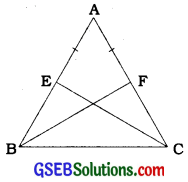In ∆ABF and ∆ACE,
AB = AC (Given)
∠A = ∠A (Common)
AF = AE (Halves of equal sides)
∴ ∆ABF ≅ ∆ACE (SAS rule)
∴ BF = CE (CPCT)

Example 3:
In an isosceles triangle ABC, with AB = AC, D and E are points on BC such that BE = CD (see the given figure). Show that AD = AE.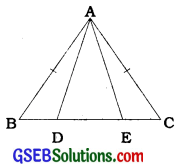In ∆ABD and ∆ACE,
AB = AC (Given) ………(1)
∠B = ∠C (Angles opposite to equal sides) …….(2)
Also, BE = CD
So, BE – DE = CD – DE
∴ BD = CE (3)
∴ ∆ABD ≅ ∆ACE
(Using (1), (2), (3) and SAS rule)
Note: The required result can also be obtained by proving ACD z ABE by SAS rule.

Some more criteria for congruence of triangles:
Theorem 7.4 (SSS congruence rule):
If three sides of one triangle are equal to the three sides of another triangle, then the two triangles are congruent.

Theorem 7.5 (RHS congruence rule):
If in two right triangles the hypotenuse and one side of one triangle are equal to the hypotenuse and one side of the other triangle, then the two triangles are congruent.
Note: RHS stands for Right angle-Hypotenuse – Side.

Example 1:
AB is a line segment. P and Q are points on opposite sides of AB such that each of them is equidistant from the points A and B (see the given figure). Show that the line PQ is the perpendicular bisector of AB.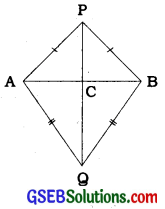PA = PB and QA = QB.
Let PQ intersect AB at C.
In ∆PAQ and ∆PBQ,
AP = BP (Given)
AQ = BQ (Given)
PQ = PQ (Common)
∆PAQ s ∆PBQ (SSS rule)
∠APQ = ∠BPQ (CPCT)

Now, in ∆PAC and ∆PBC,
AP = BP (Given)
∠APC = ∠BPC (∠APQ = ∠BPQ proved above)
PC = PC (Common)
∆PAC = ∆PBC (SAS rule)
∴ AC = BC (CPCT) ……….(1)
Moreover, ∠ACP = ∠BCP (CPCT)

But, ∠ACP + ∠BCP = 180° (Linear pair)
So, ∠ACP = 180°
or, ∠ACP = 90° … … (2)
From (1) and (2), we can easily conclude that PQ is the perpendicular bisector of AB.

Example 2:
P is a point equidistant from two lines l and m intersecting at point A (see the given figure). Show that the line AP bisects the angle between them.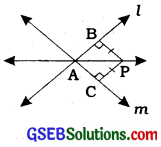Lines l and m intersect each other at A. Let PB ⊥l, PC ⊥ m. It is given that PB = PC.
In ∆PAB and ∆PAC,
PB = PC (Given)
∠PBA = ∠PCA = 90° (Given)
PA = PA (Common)
∆PAB = ∆PAC (RHS rule)
∠PAB = ∠PAC (CPCT)
Thus, line AP bisects the ∠BAC which the angle between the lines I and m.
Note: This result is the converse of the result proved in Sum no: 5 of Exercise 7.1.Inequalities in a Triangle:
Theorem 7.6:
If two sides of a triangle are unequal, the angle opposite to the longer side is larger (or greater).

Theorem 7.7:
In any triangle, the side opposite to the larger (greater) angle is longer.
Note: In a right triangle, both the angles other than the right angle are acute angles. Hence, the side opposite to the right angle, i.e., the hypotenuse is larger than each of the other two sides. Thus, in a right triangle, hypotenuse is the largest side.

Theorem 7.8:
The sum of any two sides of a triangle is greater than the third side.
By theorem 7.8, in ∆ABC, AB + BC > CA, BC + CA > AB and CA + AB > BC.
From that, the following results In the form of absolute difference can also be obtained easily:
CA > |AB – BC|, AB > |BC – CA| and
BC > |CA – AB|.
In short, In AABC,
AB + BC > CA > |AB – BC|,
BC + CA > AB > |BC – CA| and
CA + AB > BC > |CA – AB|.
Similarly, given any two sides of a triangle, the boundaries for the perimeter of the triangle can be obtained In the form of the following Inequality:
2 × (Sum of two given sides) > PerImeter of triangle > 2 × (Greater of two given sides)

Example 1:
D is a point on side BC of A ABC such that AD = AC (see the given figure). Show that AB > AD.